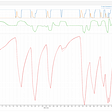# Management Accounting: Part 2: Cost Classification and behaviour:

the first task is to find the cost units in a business. for example the unit of cost for an auditing company is an hour of job, while for a builder is a unit apartment.

Production costs:

Direct Cost: identified with allocated cost unit. for example every cost which is directly related to a desk in desk making workshop is direct cost. like labour hour and the woods needed for a desk. direct cost can be measured directly by seeing the case. these two costs together are called Prime Cost.

direct cost is not the whole cost. rent cost, cost of supervision, and … are involved which are called to be Indirect cost.

• rent of factory
• Maintenance Wages
• Depreciation of Machines

Non Production costs:

• Wages of Accounts department
• rent of offices
• Delivery to customer
• or any cost which is not related to the profuction.

## Cost Behaviour:

Variable cost: the total cost varies with the level of production, eg, materials 5\$ per unit.

however the cost per unit is as below:

fixed cost doesnot vary with the level of production. like the rent of a factory.

© Stepped fixed cost: You imagine renting a factory for 10,000. but the maximum area is occupied to grow your business you need the second factory.

D. Semi variable/fixed cost: for instance electricity of a factory. we use the electricity to power the machines and light the factory. the first one is variable, while the second is fixed. so the bill payment for that is fixed variable cost.

so based on these definitions we understand that in any ways, the direct costs are variable, while the indirect costs can be variable or fixed.

Example: Variable and fixed cost:

the following is the cost schedule of a factory. please find the fixed cost.

30000= FC+n*VCperUnit= FC+200*VCperUnit

110000=FC+1000*VCperUnit

→ 80000= 800*Vcperunit → VCper unit=100

FC=10,000

Ordering and accounting for inventory:

there are three different ways for calculation of the inventory items in our warehouses.

20+140=160–80=80+100=180–140=40

1. First in first out (FIFO):

1 nov | 20|4 usd → 80USD

8 Nov| 140 |4.40 → 696

12 Nov| -80| 20 (4)+60 (4.40)

80 left which is 4.4= 352

18 Nov |100| 4.60

→ 180 : 812

26 Nov | 80 (4.4) | 60 (4.60)

→ 40*4.60 = 184

2. Last in First Out: (LIFO)

1 Nov | 20|4 usd → 80USD

8 Nov| 140 |4.40 → 696

12 Nov| -80| 80 (4.40)=352

20 left @ 4=80

60 left which is 4.4= 264

In total 80=344

18 Nov |100| 4.60 =460

→ 180 left: 804

26 Nov | 100 (4.6) | 40 (4.4)

→ 20*4.4+20*4=168

3. Weighted Average cost:

20*4+140*4.4=696/160= 4.35 per unit

remianed: 80*4.35=348

18 nov:+180*4.60=808

Average price= 4.49

40*4.49=180

Inventory control:

Ordering fixed quantities at regular intervals: like we need 12000 units per year, so we can order 12 times a year at the value of 1000 units. the graph below shows different possible combinations of the holding cost and the order quantity costs and the total cost.

the economic order quantity is the minimum cost that in which the quantity of the order and the cost of holding is optimized.

--

--

--

## More from Management Accounting

ACCA Management Accounting

## Buffer Overflow Challenge #1 Walkthrough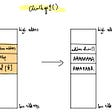## First Public Debut of xx network Code## Which SmartGlasses work best for remote collaboration? (Part 3)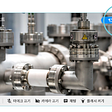## Async therefore I am## Understanding Django’s Work Flow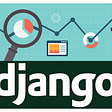## How to use Redis-Template in Java Spring Boot?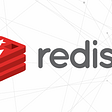## Iman Najafi

An Enthusiast Equity Analyst and Independent Financial Researcher with a passion for Fundamental Analysis. I use Medium for the daily records.

## Modelling COVID-19 with SEIR using Mathematica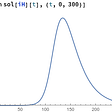## New journey## Log4j 0-Day Vulnerability: All You Need To Know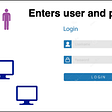## The Heart of F1